# NCERT Solutions for Class 9 Maths Chapter 7 Exercise 7.1 – Triangles

Download NCERT Solutions for Class 9 Maths Chapter 7 Exercise 7.1 – Triangles. This Exercise contains 8 questions, for which detailed answers have been provided in this note. In case you are looking at studying the remaining Exercise for Class 9 for Maths NCERT solutions for Chapter 7 or other Chapters, you can click the link at the end of this Note.

### NCERT Solutions for Class 9 Maths Chapter 7 Exercise 7.1 – Triangles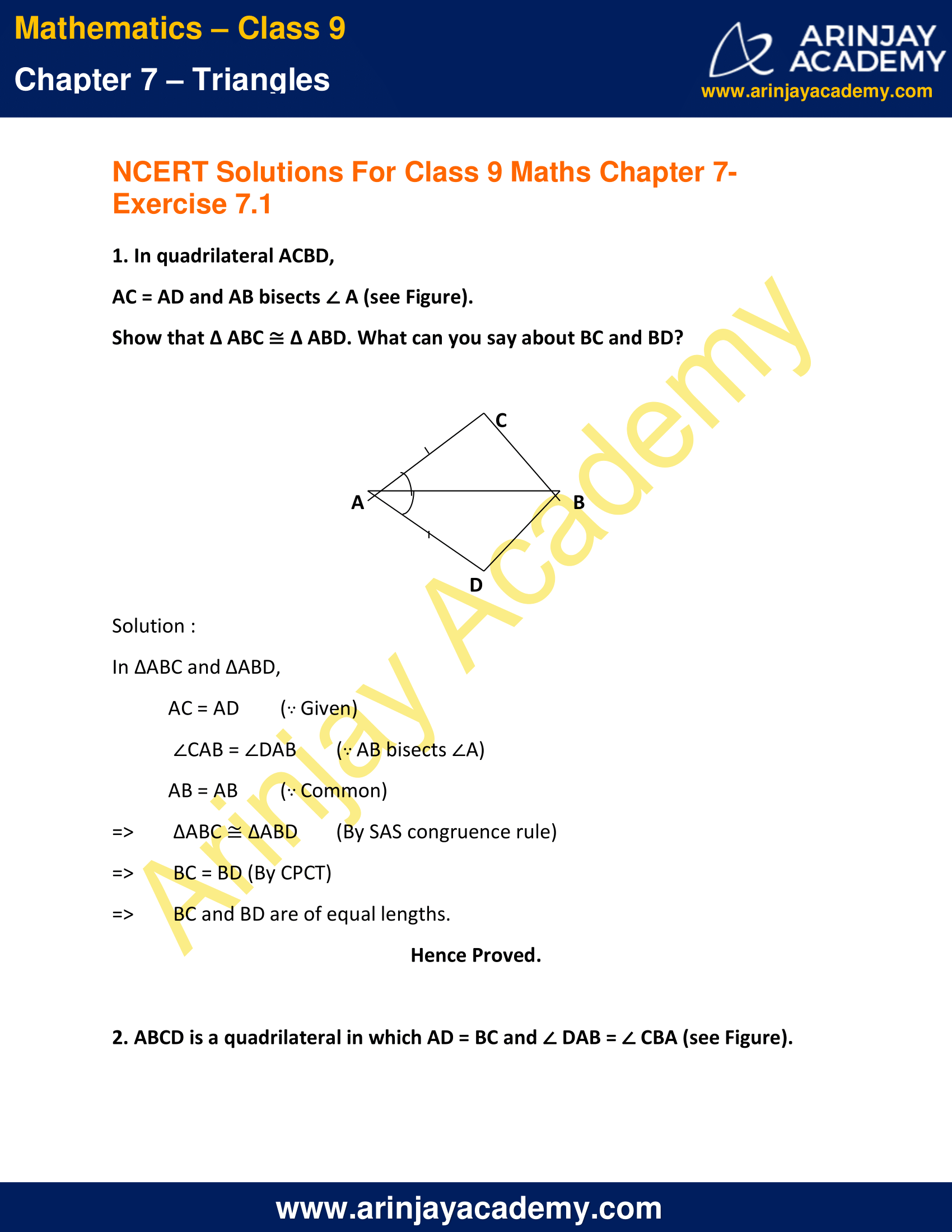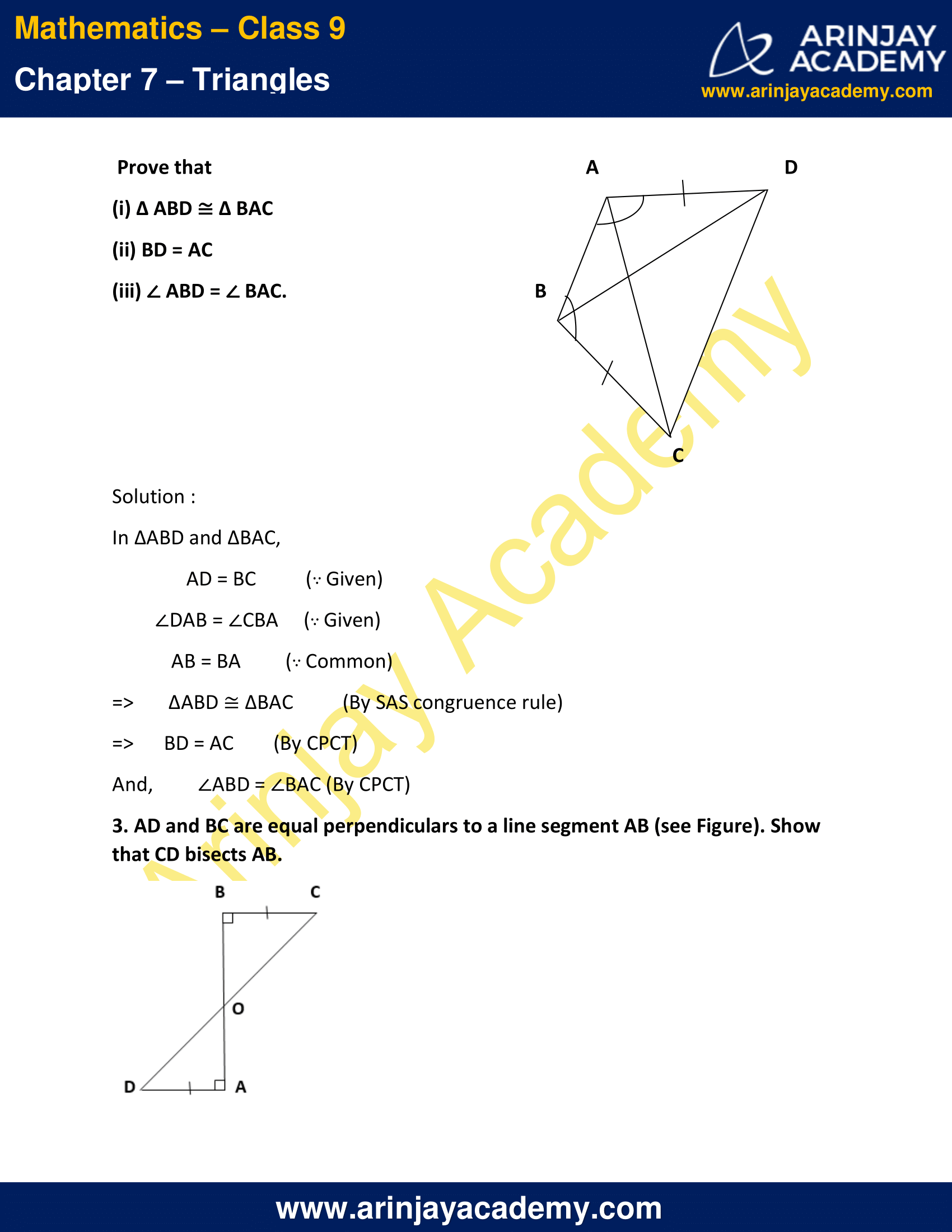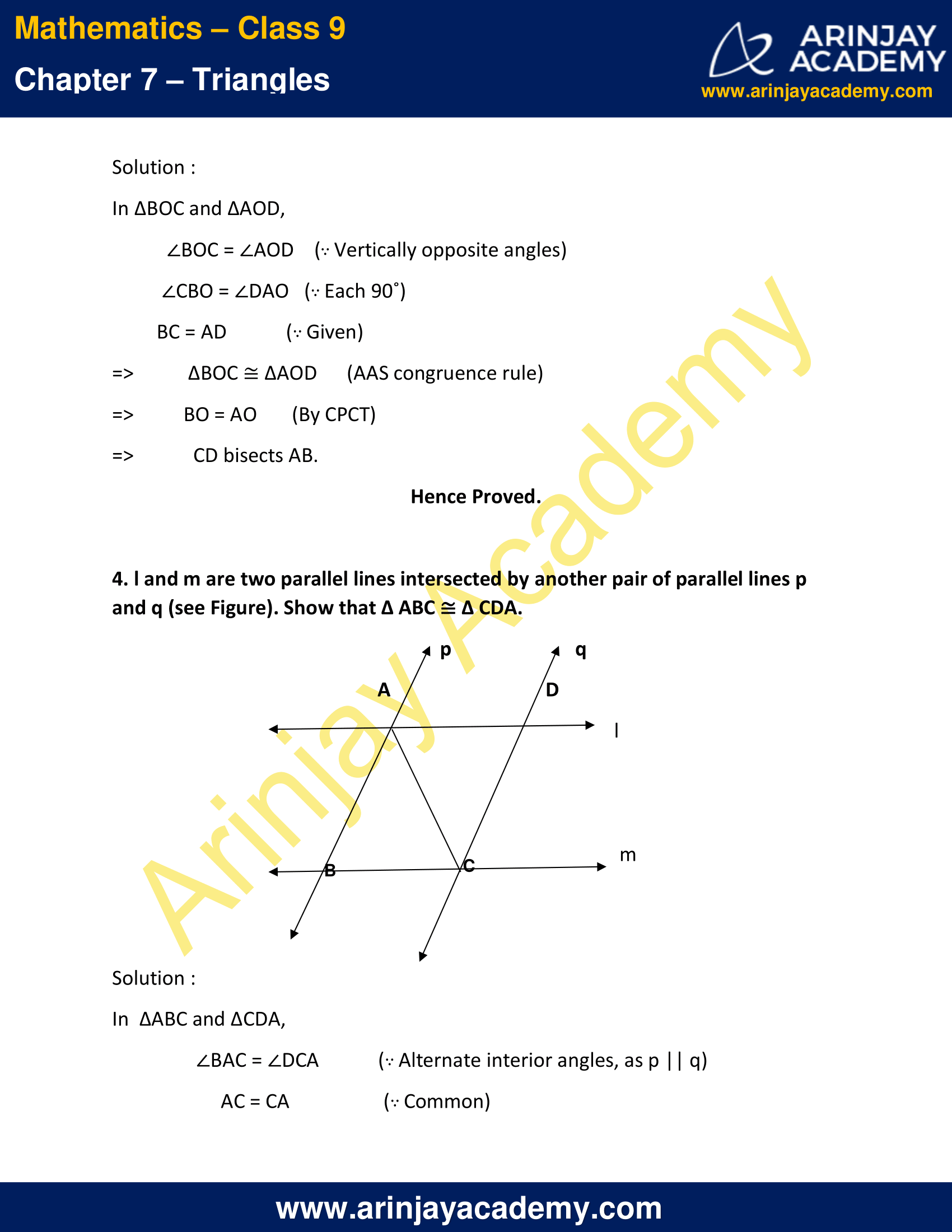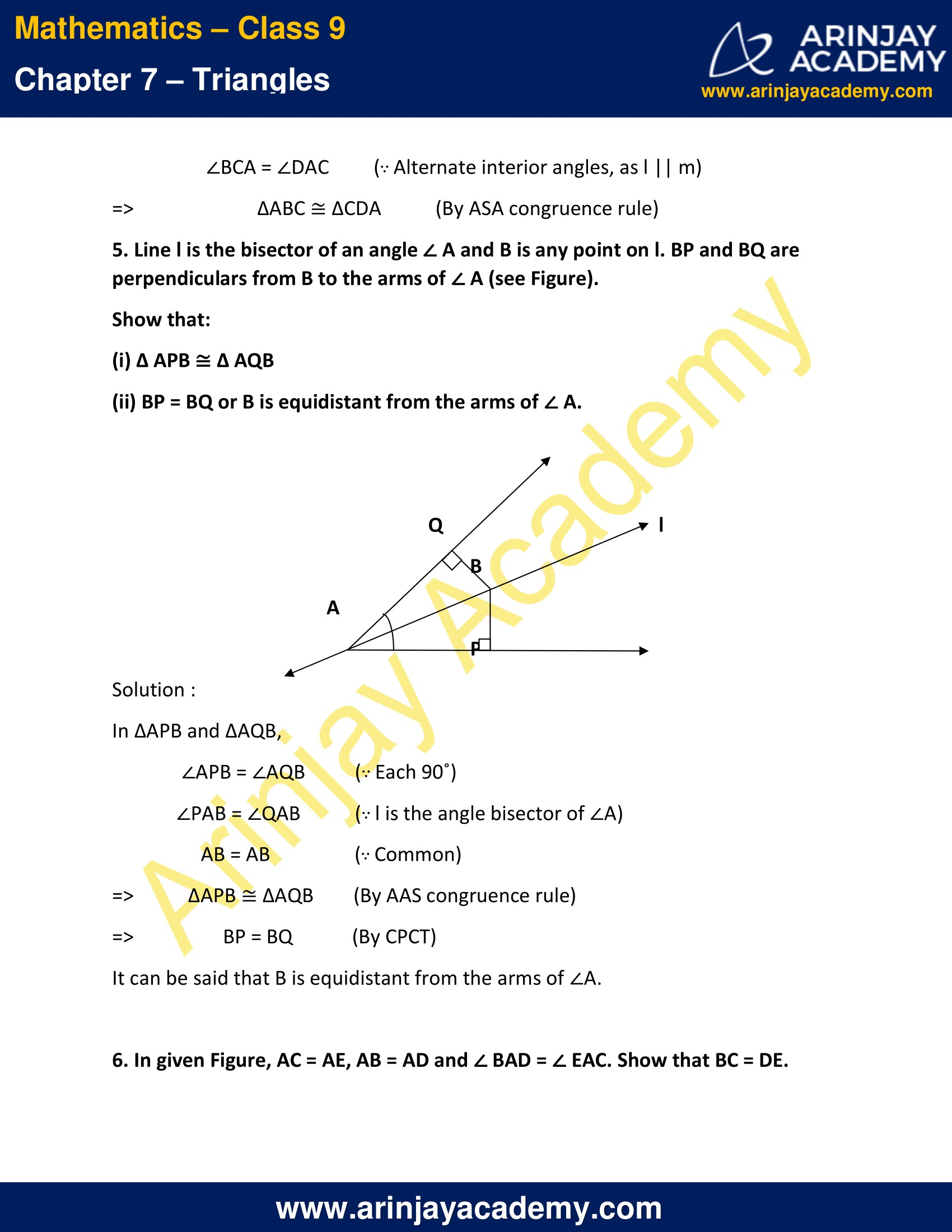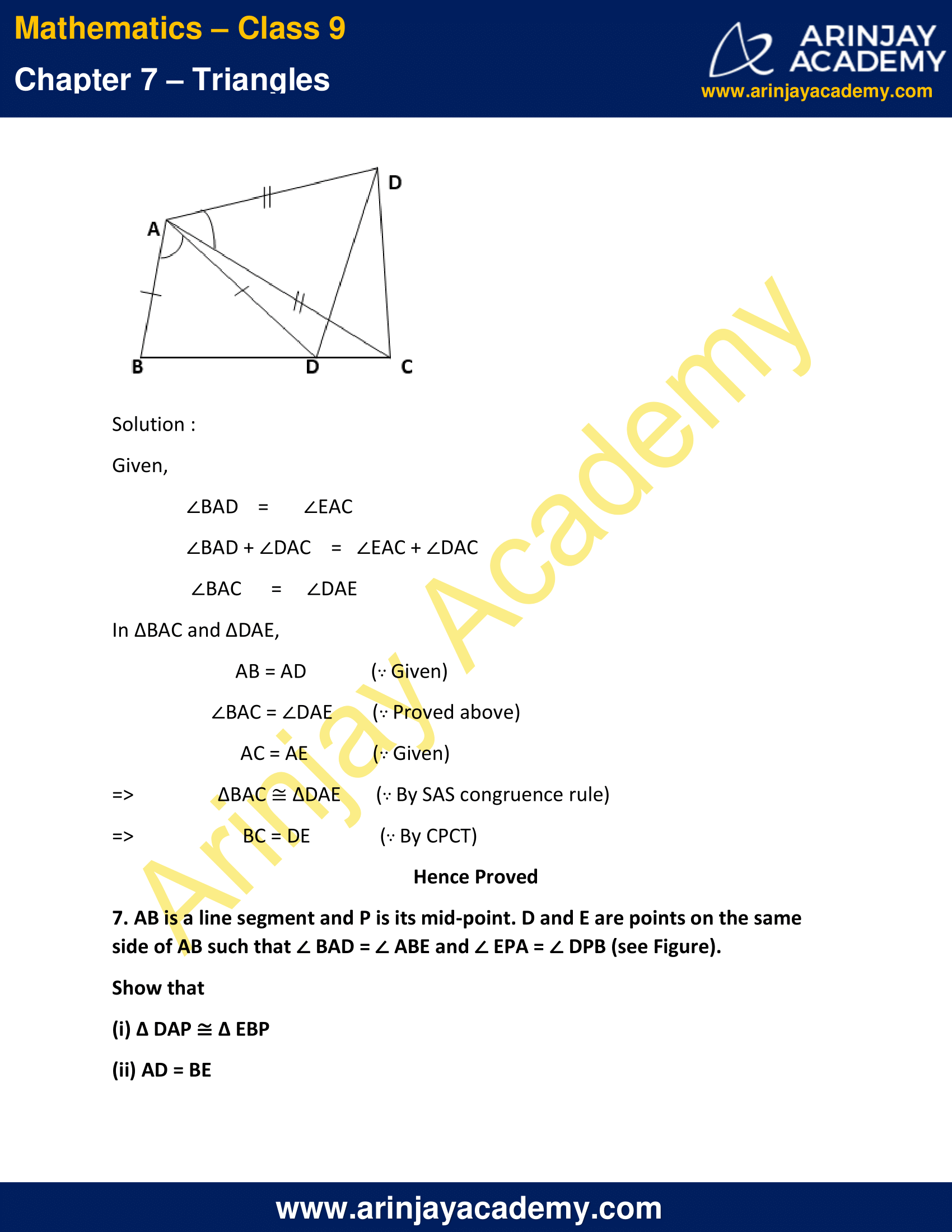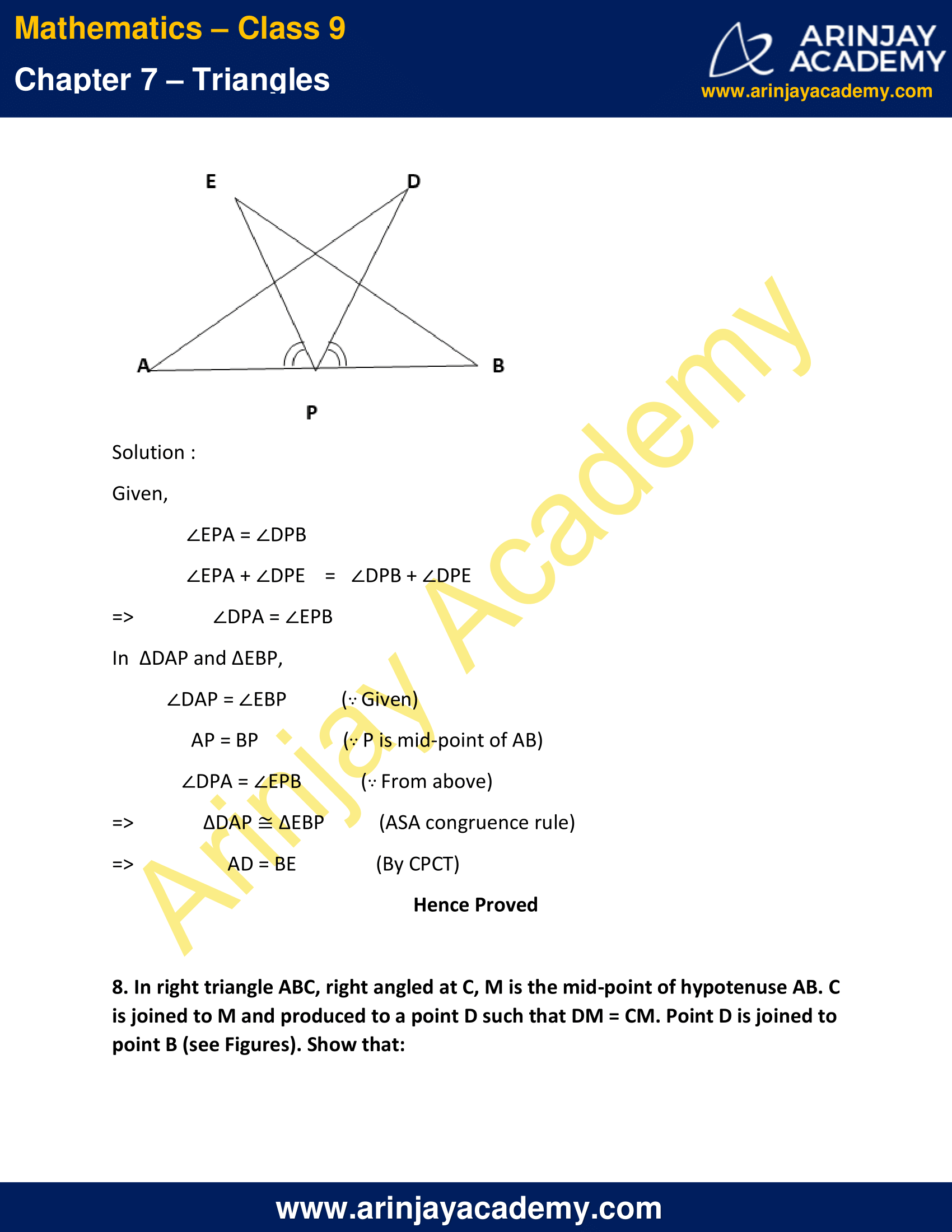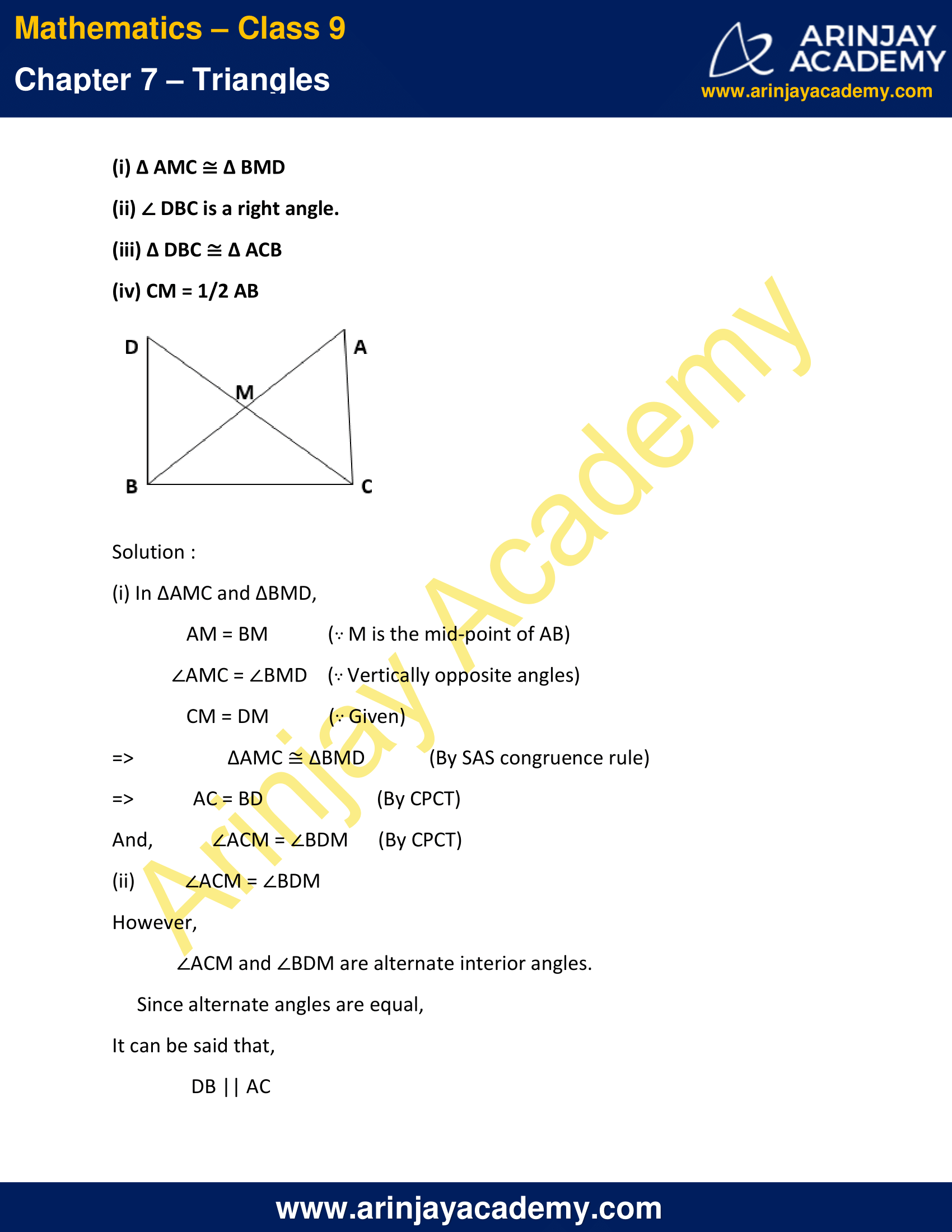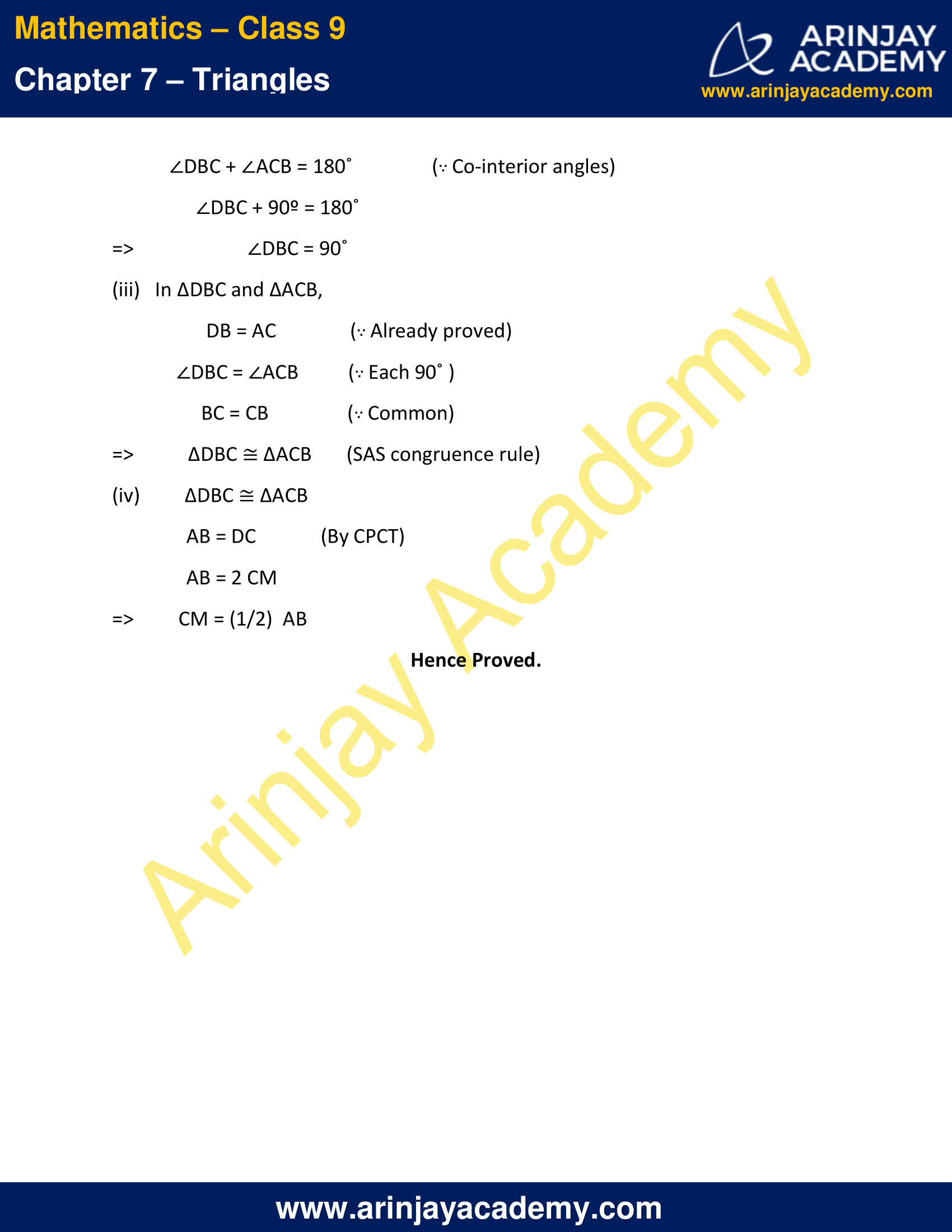NCERT Solutions for Class 9 Maths Chapter 7 Exercise 7.1 – Triangles

AC = AD and AB bisects A (see Figure).
Show that ∆ ABC ∆ ABD. What can you say about BC and BD?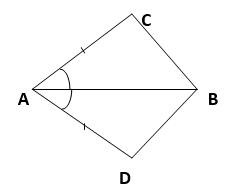Solution :

In ∆ABC and ∆ABD,
∠CAB = ∠DAB  (∵ AB bisects ∠A)
AB = AB  (∵ Common)
=> ∆ABC ≅ ∆ABD  (By SAS congruence rule)
=> BC = BD (By CPCT)
=> BC and BD are of equal lengths.

Hence Proved.

2. ABCD is a quadrilateral in which AD = BC and DAB = CBA (see Figure).
Prove that

(i) ∆ ABD ∆ BAC
(ii) BD = AC
(iii) ABD = BAC.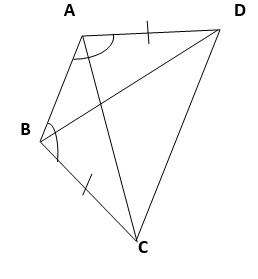Solution :

In ∆ABD and ∆BAC,
∠DAB = ∠CBA  (∵ Given)
AB = BA  (∵ Common)
=> ∆ABD ≅ ∆BAC  (By SAS congruence rule)
=> BD = AC  (By CPCT)
And, ∠ABD = ∠BAC (By CPCT)
Hence Proved.

3. AD and BC are equal perpendiculars to a line segment AB (see Figure). Show that CD bisects AB.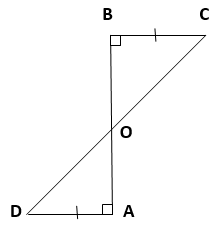Solution :

In ∆BOC and ∆AOD,
∠BOC = ∠AOD    (∵ Vertically opposite angles)
∠CBO = ∠DAO   (∵ Each 90º)
=>   ∆BOC ≅ ∆AOD   (AAS congruence rule)
=>  BO = AO  (By CPCT)
=>  CD bisects AB.
Hence Proved.

4. l and m are two parallel lines intersected by another pair of parallel lines p and q (see Figure). Show that ∆ ABC ∆ CDA.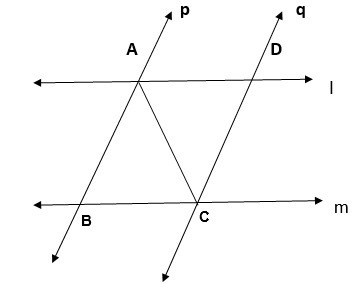Solution :

In  ∆ABC and ∆CDA,
∠BAC = ∠DCA  (∵ Alternate interior angles, as p || q)
AC = CA   (∵ Common)
∠BCA = ∠DAC  (∵ Alternate interior angles, as l || m)
=>  ∆ABC ≅ ∆CDA  (By ASA congruence rule)

5. Line l is the bisector of an angle A and B is any point on l. BP and BQ are perpendiculars from B to the arms of A (see Figure).
Show that:
(i) ∆ APB ∆ AQB
(ii) BP = BQ or B is equidistant from the arms of A.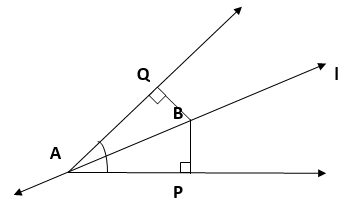Solution :

In ∆APB and ∆AQB,
∠APB = ∠AQB  (∵ Each 90º)
∠PAB = ∠QAB  (∵ l is the angle bisector of ∠A)
AB = AB   (∵ Common)
=> ∆APB ≅ ∆AQB  (By AAS congruence rule)
=> BP = BQ   (By CPCT)
It can be said that B is equidistant from the arms of ∠A.

6. In given Figure, AC = AE, AB = AD and BAD = EAC. Show that BC = DE.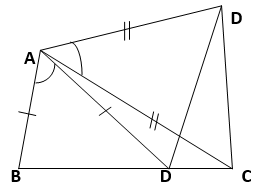Solution :

Given,
∠BAD + ∠DAC = ∠EAC + ∠DAC
∠BAC = ∠DAE
In ∆BAC and ∆DAE,
∠BAC = ∠DAE  (∵ Proved above)
AC = AE   (∵ Given)
=> ∆BAC ≅ ∆DAE   (∵ By SAS congruence rule)
=> BC = DE   (∵ By CPCT)
Hence Proved

7. AB is a line segment and P is its mid-point. D and E are points on the same side of AB such that BAD = ABE and EPA = DPB (see Figure).

Show that
(i) ∆ DAP ∆ EBP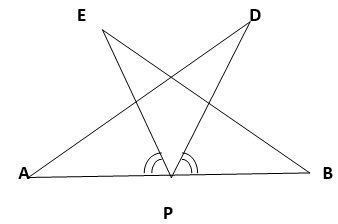Solution :

Given,
∠EPA = ∠DPB
∠EPA + ∠DPE = ∠DPB + ∠DPE
=> ∠DPA = ∠EPB
In  ∆DAP and ∆EBP,
∠DAP = ∠EBP (∵ Given)
AP = BP (∵ P is mid-point of AB)
∠DPA = ∠EPB  (∵ From above)
=> ∆DAP ≅ ∆EBP   (ASA congruence rule)
=> AD = BE    (By CPCT)
Hence Proved

8. In right triangle ABC, right angled at C, M is the mid-point of hypotenuse AB. C is joined to M and produced to a point D such that DM = CM. Point D is joined to point B (see Figures). Show that:
(i) ∆ AMC ∆ BMD
(ii) DBC is a right angle.
(iii) ∆ DBC ∆ ACB
(iv) CM = 1/2 AB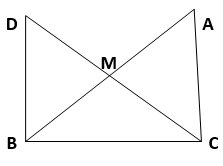Solution :

(i) In ∆AMC and ∆BMD,
AM = BM   (∵ M is the mid-point of AB)
∠AMC = ∠BMD    (∵ Vertically opposite angles)
CM = DM   (∵ Given)
=> ∆AMC ≅ ∆BMD  (By SAS congruence rule)
=>  AC = BD  (By CPCT)
And, ∠ACM = ∠BDM   (By CPCT)

(ii) ∠ACM = ∠BDM
However,
∠ACM and ∠BDM are alternate interior angles.
Since alternate angles are equal,
It can be said that,
DB || AC
∠DBC + ∠ACB = 180º  (∵ Co-interior angles)
∠DBC + 90º = 180º
=> ∠DBC = 90º

(iii)   In ∆DBC and ∆ACB,
DB = AC   (∵ Already proved)
∠DBC = ∠ACB  (∵ Each 90 )
BC = CB   (∵ Common)
=> ∆DBC ≅ ∆ACB   (SAS congruence rule)

(iv)  ∆DBC ≅ ∆ACB
AB = DC   (By CPCT)
AB = 2 CM
=>  CM = (1/2)  AB
Hence Proved.

NCERT Solutions for Class 9 Maths Chapter 7 Exercise 7.1 – Triangles, has been designed by the NCERT to test the knowledge of the student on the following topics:-

• Congruence of Triangles
• Criteria for Congruence of Triangles
– SAS congruence rule
– ASA congruence rule

The next Exercise for NCERT Solutions for Class 9 Maths Chapter 7 Exercise 7.2 – Triangles can be accessed by clicking here.

Download NCERT Solutions for Class 9 Maths Chapter 7 Exercise 7.1 – Triangles